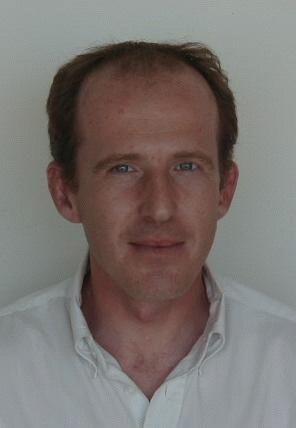# Xavier Pennec

## Geometric Statistics

In many domains, one is confronted to data which live in non-linear manifolds. For instance, the core methods of computational anatomy rely on the statistical analysis of shapes. However, statistics on non-linear spaces are more difficult than in Euclidean spaces do the non-linearities. The goal of geometric statistics is to design a consistent statistical framework on manifolds, Lie groups, and more general geometric structures.

### Simple statistics on Riemannian manifolds

The geometric structure that considered are more specially Riemannian manifolds and Lie groups. Roughly speaking, the main steps are to redefine the mean as the minimizer of an intrinsic quantity: the Riemannian squared distance to the data points. When the Fréchet mean is determined, one can pull back the distribution on the tangent space at the mean to define higher order moments like the covariance matrix. For medical image anlaysis applications, I proposed in my [PhD, 1996, (in French)] the use of the Fréchet mean and related estimation tools, which was later reformulated as an intrinsic statistical theory on manifolds.

### Manifold-valued image processing algorithms

The Fréchet mean was also the basis to generalize many algorithms to manifold-valued images on the example of diffusion tensor imaging (images of positive definite matrices), such as interpolation, filtering, diffusion and restoration of missing data.

### PCA on manifolds

My current interest is on the generalization of Principal Component Analysis (PCA) to manifolds. Tangent PCA was the natural tool used with the above statistical framework. However, if it is often sufficient for analyzing data which are sufficiently centered around a central value (unimodal or Gaussian-like data), it fails for multimodal or large support distributions (e.g. uniform on close compact subspaces). Instead of a covariance matrix analysis, Principal Geodesic Analysis (PGA) and Geodesic PCA (GPCA) are proposing to minimize the distance to Geodesic Subspaces (GS) which are spanned by the geodesics going through a point with tangent vector is a restricted linear sub-space of the tangent space. Other methods like Principal Nested Spheres (PNS) restrict to simpler manifolds but emphasize on the need for the nestedness of the resulting principal subspaces. In my work, I first propose a new and more general type of family of subspaces in manifolds, call barycentric subspaces. They are implicitly defined as the locus of points which are weighted means of k+1 reference points. As this definition relies on points and do not on tangent vectors, it can also be extended to geodesic spaces which are not Riemannian. For instance, in stratified metric spaces, it naturally allows to have principal subspaces that span over several strata, which is not the case with PGA. Barycentric subspaces locally define a submanifold of dimension k which generalizes geodesic subspaces. Like PGA, barycentric subspaces can naturally be nested, which allow the construction of inductive forward nested subspaces approximating data points which contains the Frechet mean. However, it also allows the construction of backward flags which may not contain the mean. The second contribution is to rephrase PCA in Euclidean spaces as an optimization on flags of linear subspaces (a hierarchies of properly embedded linear subspaces of increasing dimension). I propose for that an extension of the unexplained variance criterion that generalizes nicely to flags of barycentric subspaces in Riemannian manifolds. This results into a particularly appealing generalization of PCA on manifolds, that is called Barycentric Subspaces Analysis (BSA).

### Cartan-Schouten connections and Bi-invariant means on Lie groups

In order to obtain a statistical framework which is fully compatible with Lie groups (bi-invariance), I propose to replace the right-invariant Riemnanian metric setting by the canonical Cartan connection on the group. The geodesics are translations of one-parameter subgroups and the mean value is extended to such a non-metric structure using an implicit definition: the exponential barycenter. With Nina Miolane, we investigated the relaxation of the positivity of the metric in order to obtain consistent statistics on Lie groups through a bi-invariant pseudo-Riemannian framework. Unfortunately, most of the commonly used Lie groups do not admit a bi-invariant pseudo-Riemannian metric either.

### The Stationary Velocity Fields (SVF) framework for diffeomorphisms

The extension of the Cartan-Schouten framework to infinite dimensions grounds the parameterization of (a subset of) diffeomorphisms by the flow of Stationary Velocity Fields (SVFs). One-parameter subgroups are now used in numerous non-linear medical image registration algorithms. This idea was introduced by Arsigny in 2006, but fully understood as the geodesics of the Cartan-Schouten connection in 2013. This reformulation allowed to propose dicrete parallel transport techniques based on the Schild ladder to transport deformation trajectories from one subject to the reference template. For that purpose, we have proposed with M. Lorenzi an algorithm based on the Schild’s ladder to realize the parallel transport along any curve

Xavier Pennec